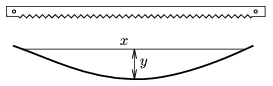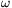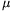Mathematical and Physical Journal
for High Schools
Issued by the MATFUND Foundation
 Already signed up? New to KöMaL?

# KöMaL Problems in Physics, March 2006

Show/hide problems of signs:## Problems with sign 'M'

Deadline expired on April 11, 2006.

M. 268. The blade of a simple hacksaw is usually about 3 cm long and at its ends there are small holes. Connect the two ends of the blade with pieces of strong strings of different lengths. How does the bending of the blade (y) depend on the distance between the holes x? Measure how does the tension in the string depends on the distance x.(6 pont)

statistics## Problems with sign 'P'

Deadline expired on April 11, 2006.

P. 3874. Explain how a level works. Instead of a level can we use any tube filled with water and having a small bubble in it?

(3 pont)

statistics

P. 3875. Why does the refrigerator turn on more times in summer than in winter?

(3 pont)

statistics

P. 3876. You can barely hear if you clap your hands in knitted gloves. Why? What causes the sound when you are clapping?

(4 pont)

statistics

P. 3877. The planes in airports are pulled by small lorries loaded by heavy concrete blocks. What is the greatest acceleration of a lorry of mass m if it pulls a plane of mass 70 tons. Find the least mass of the lorry which is able to move a plane of mass 70 tons, if the coefficient of static friction on wet concrete is 0.4 and on dry concrete is 0.8, and the coefficient of rolling friction is 0.02.

(4 pont)

statistics

P. 3878. A right circular cone shaped funnel rotates about its vertical symmetry axis at an angular speed of=10 s-1. What is the position of that small object which rotates together with the funnel? Data:0=0.2; half of the vertex angle of the cone 30o.

(4 pont)

statistics

P. 3879. One end of a chord is attached to a small body which undergoes circular motion on a horizontal frictionless table. There is a hole on the table at the centre of the circle, the chord goes through the hole and the other end of the chord is held by our hand. Pulling this end the body moves from the path of radius r1 to another path of radius r2. By what factor does the kinetic energy of the body change? By what factor does the tension change?

(4 pont)

statistics

P. 3880. A space-shuttle revolves around the Earth along an elliptical path, the smallest distance between the Earth and the shuttle is 6870 km (measured from the centre of the Earth), the speed of the shuttle at this position is 10 km/s. a) What is the greatest distance between the shuttle and the Earth (measured from the centre of the Earth)? b) What is the least speed of the shuttle?

(4 pont)

statistics

P. 3881. Two similar inlined planes are attached to each other such that their edges at the bottom touch each other. A small uniform disk of radius r is placed to the inclined planes horizontally. The disk is moved out of its equilibrium position, and begins to oscillate without slipping. Find the period of the motion.

(5 pont)

statistics

P. 3882. There is a sample of diatomic gas in a container (whose volume is constant). The temperature of this gas was increased greatly so some molecules fell into atoms (dissociated) and the pressure of the gas increased by a factor of 9.2 and the internal energy of the gas increased to a value of 8.24 times the original internal energy. a) What percent of the molecules dissociated? b) By what factor did the temperature of the gas (measured in Kelvins) increased?

(4 pont)

statistics

P. 3883. During the fission neutrons are ejected with an initial speed of 2.107 m/s. We would like to slow down these neutrons in heavy water to a speed of 103 m/s. At least how many collisions must occur?

(4 pont)

statistics